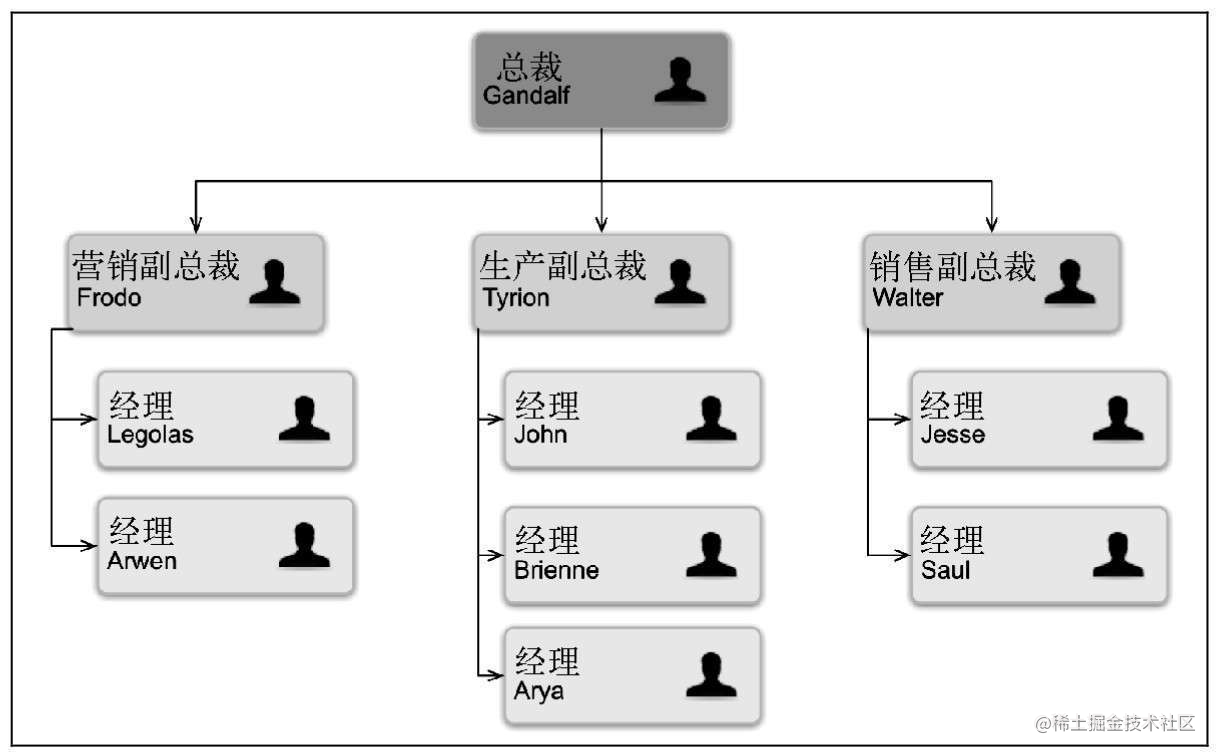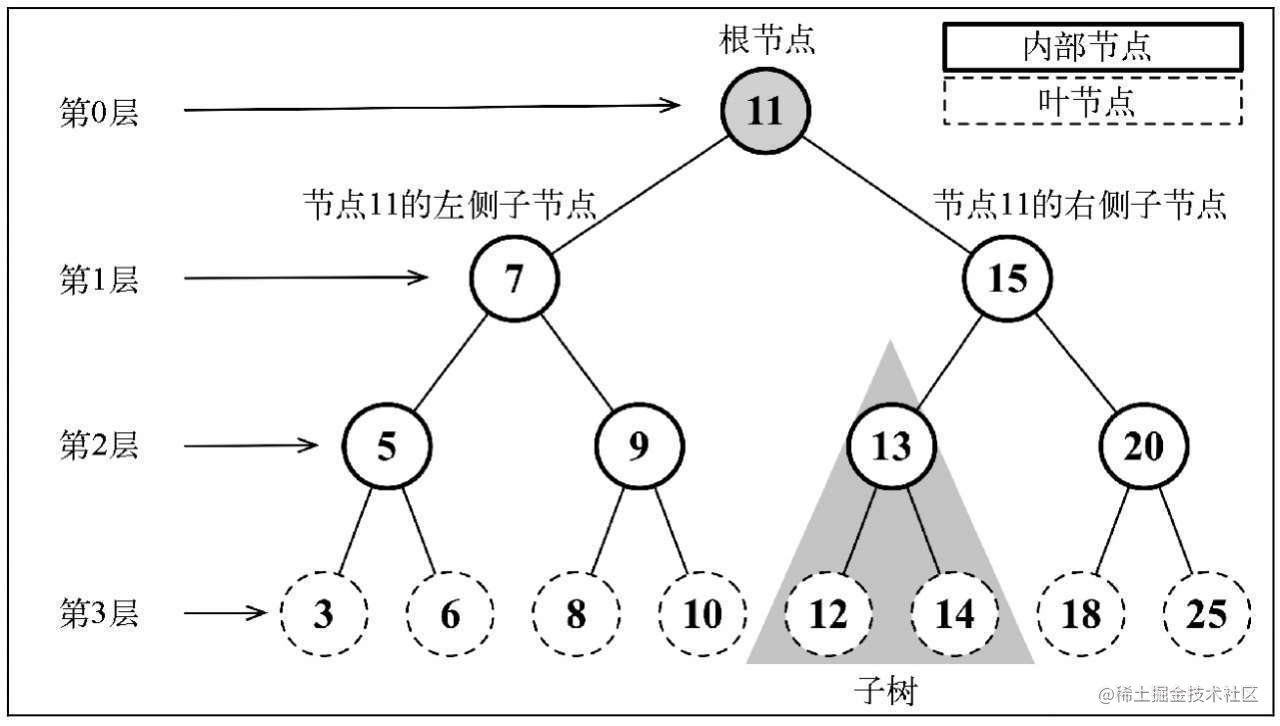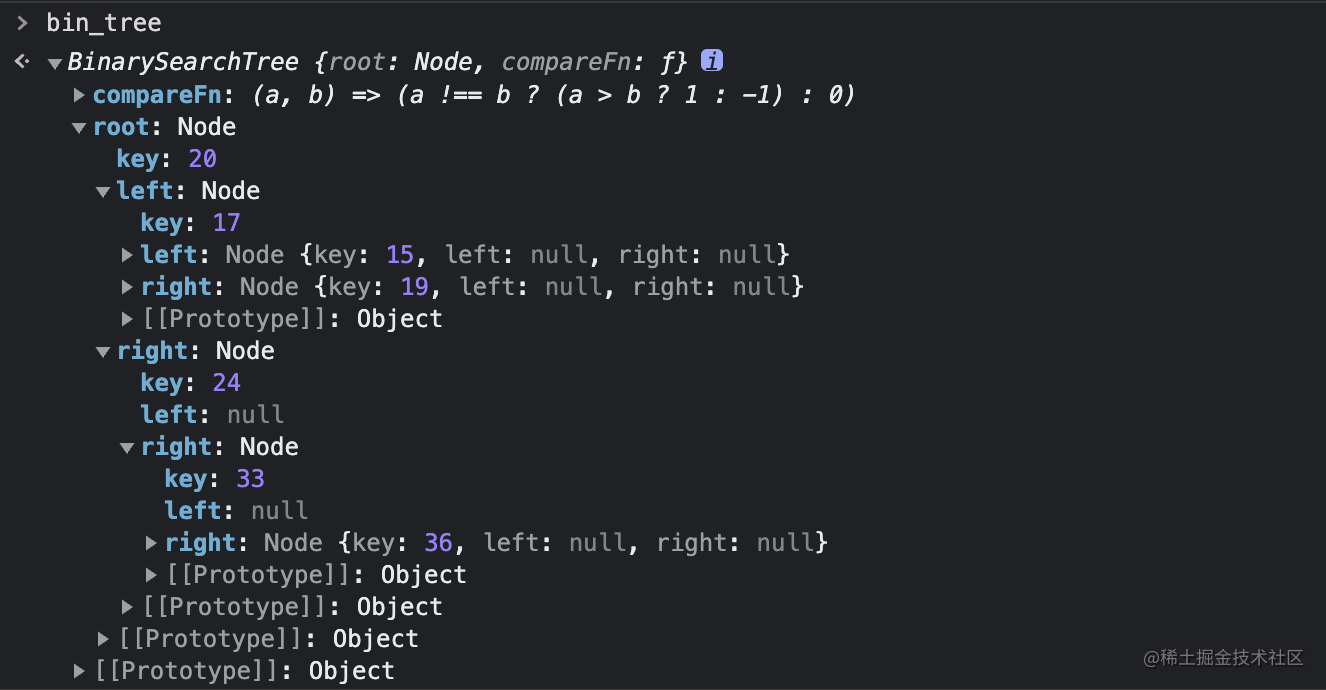# 树与二叉树## 树的相关术语• 父节点
• 兄弟节点
• 子节点

• `高度`：从 0 开始，一共有多少层
• `深度`：某个节点处在第几层

## 二叉树与二叉搜索树

`二叉搜索树`（BST）是二叉树的一种，但它要求必须在左侧子节点存储比父节点小的值，在右侧子节点存储比父节点大的值。上图中灰色部分由 13、12、14 三个节点组成的树就是一棵二叉搜索树。

### 初始化类

`class Node {  constructor(key) {    this.key = key;    this.left = null;    this.right = null;  }}`

`class BinarySearchTree {  constructor(compareFn) {    let defFn = (a, b) => (a !== b ? (a > b ? 1 : -1) : 0);    this.root = null;    this.compareFn = compareFn || defFn;  }}`

• `insert(key)`：插入一个键
• `search(key)`：在树中查找一个键
• `inOrderTraverse()`：通过中序遍历方式遍历所有节点
• `preOrderTraverse()`：通过先序遍历方式遍历所有节点
• `postOrderTraverse()`：通过后序遍历方式遍历所有节点
• `min()`：返回树中最小的值
• `max()`：返回树中最小的值
• `remove()`：从树中移除某个键

## insert 方法

insert 方法的作用是向二叉搜索树中插入一个 key（节点）。本篇的 insert 方法要比前几篇实现的复杂一些，因为会用到很多递归。这也是为什么我们在学习树之前先要介绍递归。

`insert(key) {  if(this.root == null) {    this.root = new Node(key)    return true  } else {    return this.insertNode(this.root, key)  }}`

`insertNode(node, key) {  let compare = this.compareFN(key, node.key)  if(compare < 0) {    if(node.left == null) {      node.left = new Node(key)      return true    } else {      this.insertNode(node.left, key)    }  }  if(compare > 0) {    if(node.right == null) {      node.right = new Node(key)      return true    } else {      this.insertNode(node.right, key)    }  }  return false}`

`var bin_tree = new BinarySearchTree();bin_tree.insert(20);`

`bin_tree.insert(17);bin_tree.insert(15);bin_tree.insert(24);bin_tree.insert(33);bin_tree.insert(19);bin_tree.insert(36);`